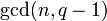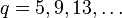# Element structure of projective general linear group of degree four over a finite field

## Contents

This article gives specific information, namely, element structure, about a family of groups, namely: projective general linear group of degree four.
View element structure of group families | View other specific information about projective general linear group of degree four

This article discusses the element structure of the projective general linear group of degree four over a finite field.

## Conjugacy class structure

### Number of conjugacy classes

As we know in general, number of conjugacy classes in projective general linear group of fixed degree over a finite field is PORC function of field size, the degree of this PORC function is one less than the degree of matrices, and we make cases based on the congruence classes modulo the degree of matrices. Thus, we expect that the number of conjugacy classes is a PORC function of the field size of degree 4 - 1 = 3, and we need to make cases based on the congruence class of the field size modulo 4. Moreover, the general theory also tells us that the polynomial function of$q$ depends only on the value of$\operatorname{gcd}(n,q-1)$, which in turn can be determined by the congruence class of$q$ mod$n$ (with$n = 4$ here).

Value of$\operatorname{gcd}(4,q-1)$ Corresponding congruence classes of$q$ mod 4 Number of conjugacy classes (polynomial of degree 4 - 1 = 3 in$q$) Additional comments
1 0 or 2 mod 4 (e.g.,$q = 2,4,8,\dots$)$q^3 + q^2 + q = q(q^2 + q + 1)$ In this case, we have an isomorphism between linear groups when degree power map is bijective, so$SL(4,q) \cong PGL(4,q) \cong PSL(4,q)$
2 3 mod 4 (e.g.,$q = 3,7,11,\dots$)$q^3 + q^2 + 2q + 1$
4 1 mod 4 (e.g.,$q = 5,9,13,\dots$)$q^3 + q^2 + 2q + 3$